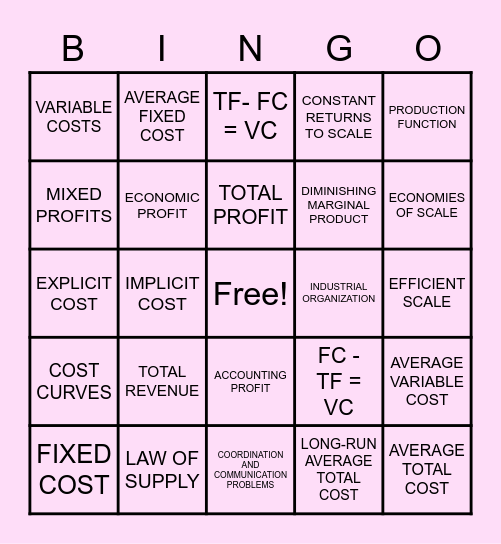# ECON BINGOThis bingo card has a free space and 29 words: FIXED COST, MINIMUM RATE OF RETURN, IMPLICIT COST, QUANTITY OUPUT, VARIABLE COSTS, TF- FC = VC, ECONOMIC PROFIT, TOTAL REVENUE, INDUSTRIAL ORGANIZATION, LAW OF SUPPLY, EFFICIENT SCALE, AVERAGE TOTAL COST, COORDINATION AND COMMUNICATION PROBLEMS, LONG-RUN AVERAGE TOTAL COST, ECONOMIES OF SCALE, MARGINAL PRODUCT, ACCOUNTING PROFIT, COST CURVES, PRODUCTION FUNCTION, EXPLICIT COST, FC - TF = VC, DISECONOMIES OF SCALE, DIMINISHING MARGINAL PRODUCT, MIXED PROFITS, CONSTANT RETURNS TO SCALE, AVERAGE FIXED COST, AVERAGE VARIABLE COST, TOTAL PROFIT and TOTAL COST.

## Play Online